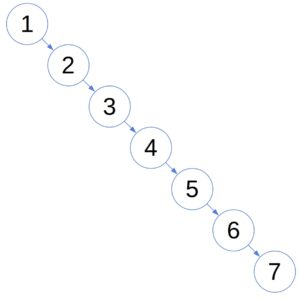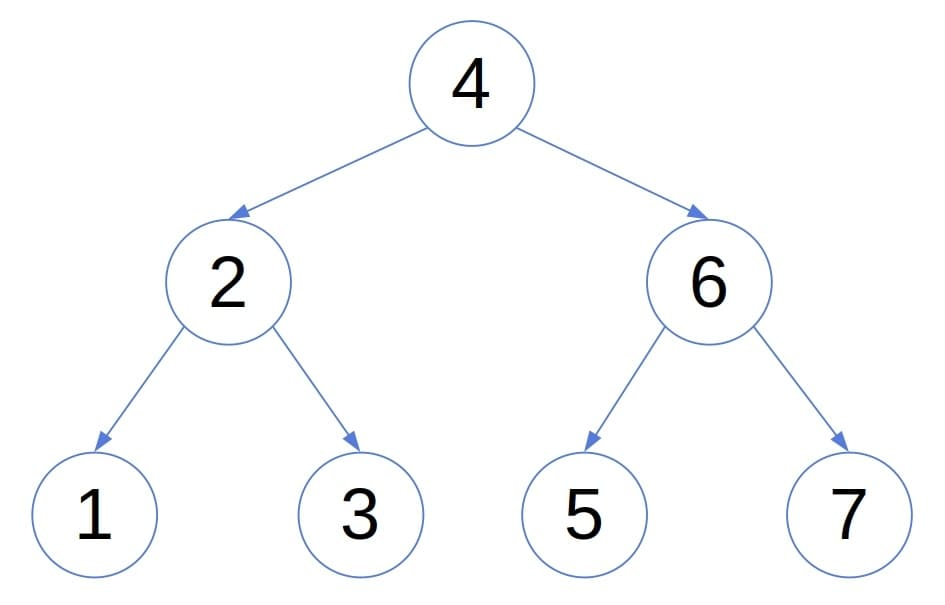## 1. Introduction

In this tutorial, we’ll explain the difference in time complexity between binary-search and AVL trees. More specifically, we’ll focus on the expected and worst-case scenarios for constructing the trees out of arrays.

When it comes to space complexity, both trees requirespace to be constructed regardless of the case analyzed.

## 2. Binary Search Trees and AVL Trees

In a binary search tree (BST), each node’s value isthan its left descendants’ values andthan the values in its right sub-tree.

The goal of BSTs is to allow efficient searches. Although that’s mostly the case, the caveat is that a BST’s shape depends on the order in which we insert its nodes. In the worst case, a plain BST degenerates to a linked list and has ansearch complexity:Balanced trees were designed to overcome that issue. While building a balanced tree, we maintain the shape suitable for fast searches. In particular, when it comes to AVL trees, the heights of each node’s left and right sub-trees differ at most by 1:That property implies that an AVL tree’s height is. To ensure it, we need to re-balance the tree if an insertion unbalances it, which incurs computational overhead.

Here, we’ll analyze the complexity of building an AVL tree and a BST out of an arrayof comparable objects:## 3. The Worst-Case Complexity Analysis

Let’s first analyze the worst-case scenario. For both BST and AVL trees, the construction algorithms follow the same pattern:The algorithm starts with an empty tree and inserts the elements ofsuccessively until processing the whole array. To place, the algorithm first looks for it in the tree containing. The search procedure identifies the nodewhose childshould become. Then, the algorithm insertsas the left or right child ofdepending on whetheror.

### 3.1. Constructing a BST

In the worst-case scenario, when the insertion orderskews the tree, the insertion into a BST is a linear-time operation.

Since the tree is essentially a list, addingto the tree in the worst case requires traversing all the previously attached elements:. In total, we followpointers:

(1)So, constructing a BST takes a quadratic time in the worst case.

### 3.2. Constructing an AVL Tree

Insertion into an AVL tree takes log-linear time. The reason is that an AVL tree’s height is logarithmic in the number of nodes, so we traverse no more thanedges when insertingin the worst case. In total:

(2)The worst-case complexity of building an AVL tree is. So, although insertions can trigger re-balancing, an AVL tree is still faster to build than an ordinary BST.

## 4. The Expected Complexity

In the following analyses, we assume that allinsertion orders ofare equally likely.

### 4.1. Binary Search Trees

A single insertion’s complexity depends on the average node depth in a BST. We can calculate it as the sum of the nodes’ depths divided by the number of nodes ().

If the root’s left sub-tree hasnodes, the right one containsvertices. Then, the expected sum of the depths in such a BST,, is:

(3)We addbecause the root node increases the depths of all the other nodes by 1.

Since all permutations ofare equally probable,follows the uniform distribution over. So, the expected depth of a node in a BST withnodes is:

(4)Solving the recurrence, we get. So, the expected node depth in a BST withnodes is. Hence, starting from an empty tree and inserting, we performsteps:

(5)Therefore, in the average case, the construction of a BST takes a log-linear time.

### 4.2. AVL Trees

Since the AVL trees are balanced and do not depend on the insertion order, the tree depth is always. Moreover, an AVL tree has at mostnodes at depth. So, the average node depth is bounded by:

(6)Since:the expected node depth in an AVL tree is:

(7)Since the average node depth is the same as in a BST, Equation (5) holds for AVL trees as well. So, building an AVL tree is also a log-linear operation in the expected case.

## 5. Conclusion

In this article, we compared the construction complexities of Binary Search Trees (BSTs) and AVL trees. In the worst-case scenario, building an AVL tree takestime, whereas constructing a BST has ancomplexity.

However, both trees take a log-linear time in the expected case.